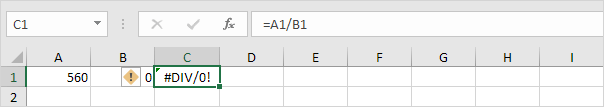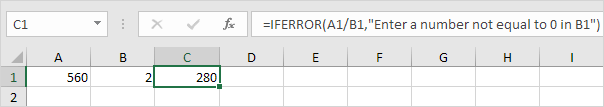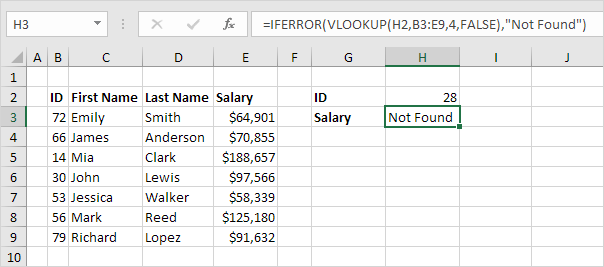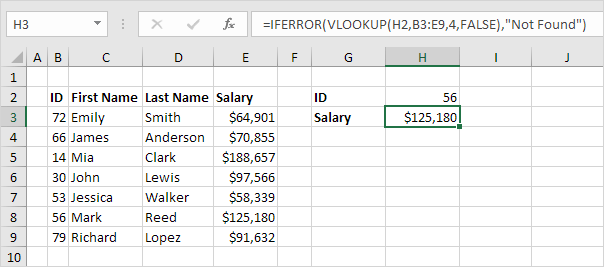# IFERROR

Use the IFERROR function in Excel to return an alternative result, such as text, when a formula evaluates to an error.

1. For example, Excel returns the #DIV/0! error when a formula tries to divide a number by 0.2. If the formula evaluates to an error, the IFERROR function below returns a friendly message.3. If the formula does not evaluate to an error, the IFERROR function simply returns the result of the formula.4. For example, Excel returns the #N/A error when the VLOOKUP function cannot find a match.5. If the VLOOKUP function evaluates to an error, the IFERROR function below returns a friendly message.6. If the VLOOKUP function does not evaluate to an error, the IFERROR function simply returns the result of the VLOOKUP function.Note: the IFERROR function catches the following errors: #DIV/0!, #N/A, #VALUE!, #REF!, #NUM!, #NAME? and #NULL!. For example, the IFERROR function shown above also catches the #NAME? error if you accidentally misspell the word VLOOKUP. Use the IFNA function in Excel 2013 or later to only catch #N/A errors.

Go to Next Chapter: Array Formulas